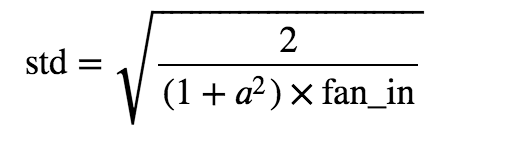# Why do we need to specify non-linearity while initializing the weights of convolutional layers with kaiming He initialization?

Hi guys, I am initializing the weights of CNN with kaiming he initializer but I dont understand why we have to specify the type of non-linear activation function in `nn.init.kaiming_normal_(m.weight, mode='fan_out', nonlinearity='relu')` . I am comfused about this non-linearity parameter, does it mean all the layers which I am initializing with kaiming he weights will have ‘relu’ as their activation function or it has something to do with the initialization itself?

@samra-irshad, If you look at the equation proposed to calculate the standard deviation by he et al, in the paper https://arxiv.org/abs/1502.01852.where a = the negative slope of the rectifier used after this layer.
So I guess this to initialize the distribution, where mean is zero and std is calculated using the above formula.
https://pytorch.org/docs/stable/modules/torch/nn/init.html#kaiming_uniform

1 Like

So does it mean the layers I initialize using `nn.init.kaiming_normal_(m.weight, mode='fan_out', nonlinearity='relu')` are all going to have `ReLU` as their activation function? In this case, I have to exclude the last layer of my model from being initialized through this method that is going to map probabilities over classes via `softmax`

I don’t think that is the case, as I mentioned above it calculates the standard deviation of the weights considering the next non-linearity which has to be applied, It doesn’t apply relu to them.
You should check the implementation in pytorch, I have shared the link above.
Take this example,

``````w = torch.empty(3, 5)
nn.init.kaiming_normal_(w, mode='fan_out', nonlinearity='relu')
>>tensor([[-0.4137,  0.3216,  0.0705, -0.4403,  0.4050],
[-0.5409,  0.3364, -0.7153,  0.2617,  0.5652],
[ 0.2512,  0.6643, -0.9265, -0.2095, -0.9202]])

``````

If you look at the above output, you’ll see a lot of negative’s as weights, which if the ReLU was applied will not be the case.

1 Like